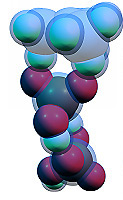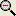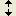## MO energies diagram window

At this window the energies of molecular orbitals from the output file are visualized in the form of diagram. There are always both occupied and unoccupied orbitals in the computation, and the occupancy of each orbital determines the color of corresponding horizontal line on the diagram. The checkbox "Show vertical arrows" enables drawing of arrows, which represent alpha and beta electrons occupying orbitals.

The buttonunzooms the diagram, the buttonpartially unzooms it (it increases the interval of Y axes).

For restricted closed-shell computations (e.g. RHF, RMP2, RB3LYP, etc), each molecular orbital is either occupied by two electrons (alpha and beta) or unoccupied.

For unrestricted computations (e.g. UHF), there are two sets of orbitals (alpha and beta), with each orbital either occupied by one electron (correspondingly alpha or beta electron) or unoccupied.

For restricted-open computations (e.g. ROHF), there is one set of orbitals, and each orbital can be occupied by two electrons, occupied by only one alpha-electron, or unoccupied.

Chemcraft calculates the difference between energies of two selected orbitals. By default, the HOMO-LUMO energy gap is calculated (the difference between the energies of lowest unoccupied orbital and highest occupied orbital; for unrestricted computations, each of these orbital can be found either in the alpha or beta set).

BACK